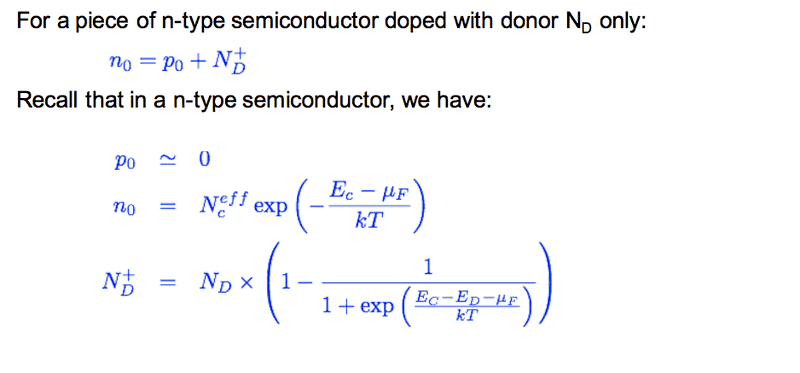# Law of Mass Action

In a semiconductor the law of mass action states that the product of the electron concentration and the hole concentration is always equal to the square of the intrinsic carrier concentration (at a given temperature), i.e.:

$$n p = n_i^2$$

My book states that this law is valid for extrinsic semiconductors (with impurities) as well as for intrinsic semiconductors. I dont understand how it can be valid for extrinsic semiconductors. In an intrinsic semiconductor charge neutrality requires n=p. I understand that the law is valid for intrinsic semiconductors. But if I start out with an intrinsic semiconductor and put in some electron donors, only $$n$$ will increase, $$p$$ and $$n_i$$ will not change. So how can it be valid in the extrinsic case?

Defennder
Homework Helper
That's because of how n and p are given in the mathematical expression.

Using the effective density of states approximation for n and p in the conduction and valence band respectively:

$$n = N_C exp(-\frac{E_C - E_F}{kT}) \ \mbox{and} \ p = N_V exp(-\frac{E_F - E_V}{kT})$$

Multiplying them together gives $$np=N_C N_V exp(-\frac{E_g}{kT})$$ since $$E_C - E_V$$ is equivalent to Eg, the bandgap.

Note that the expression on the right is independent of Ef, the fermi level and hence independent of doping. This is of course valid only under the assumption that Nc and Nv is given at some constant temperature.

But if I start out with an intrinsic semiconductor and put in some electron donors, only $$n$$ will increase, $$p$$ and $$n_i$$ will not change

That's not true at equilibrium. $$n$$ will increase initially, but after a few moments some electrons added will fall in valence band, reducing holes concentration. mass action law rules matter flow between two (classical) systems at equilibrium

Thank you for the answers! It makes more sense to me now.

I still don't understand this. Can anyone please elaborate?
What I understand is, Intrinsic carriers are the are electron hole pairs formed without doping, after doping the number of majority carriers is increased by a large number. Now how can the law holds true??

I was asked why the law of mass action in not valid at very low temperatures. I thought that it was, since for very low temperature it holds the condition $$E_g >> k_B T$$ (I think so...). But maybe for some other reason it breaks. Does someone know why???

Boltzmann statistics as a high temperature approximation of Fermi-Dirac statistics?

In a semiconductor the law of mass action states that the product of the electron concentration and the hole concentration is always equal to the square of the intrinsic carrier concentration (at a given temperature), i.e.:

$$n p = n_i^2$$

My book states that this law is valid for extrinsic semiconductors (with impurities) as well as for intrinsic semiconductors. I dont understand how it can be valid for extrinsic semiconductors. In an intrinsic semiconductor charge neutrality requires n=p. I understand that the law is valid for intrinsic semiconductors. But if I start out with an intrinsic semiconductor and put in some electron donors, only $$n$$ will increase, $$p$$ and $$n_i$$ will not change. So how can it be valid in the extrinsic case?
The rate of recombination is R=Bnp and increasing the n would increase recombination rate so that both n and p decrease and their product remains constant.

Realise this is an old thread, but I am similarly confused. If I have a ##pn## junction, then on the ##n## side of the junction, it's doped by ##N_{D}##, the p by ##N_{A}##. ##N_{D}## and ##N_{A}## (within operating temperature) should not depend on the temperature ##T##.

So how come my solution sheet says that:

$$n_{n} = N_{C} exp\bigg( -\big[\frac{ E_{C} - E_{Fn}}{k_{B}T} \big] \bigg) =N_{D}$$

##E_{Fn}## is the intrinsic fermi level on the ##n## side of the junction, and ##E_{C}## the conduction band energy.

I think that perhaps this equation from my notes might help - but it's been derived from nowhere, and I don't understand it... Would really appreciate some help on this! Thanks•quixote